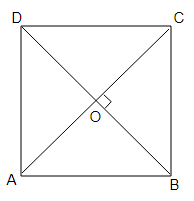# $\mathrm{ABCD}$ is a rhombus. Show that diagonal $\mathrm{AC}$ bisects $\angle \mathrm{A}$ as well as $\angle \mathrm{C}$ and diagonal $\mathrm{BD}$ bisects $\angle \mathrm{B}$ as well as $\angle \mathrm{D}$.

Given:

$\mathrm{ABCD}$ is a rhombus.

To do :

We have to show that diagonal $\mathrm{AC}$ bisects $\angle \mathrm{A}$ as well as $\angle \mathrm{C}$ and diagonal $\mathrm{BD}$ bisects $\angle \mathrm{B}$ as well as $\angle \mathrm{D}$.

Solution :$AC$ and $BD$ are the diagonals which intersect each other at $O$.

$AD = CD$     (Sides of a rhombus are equal)

$\angle DAC = \angle DCA$         (Angles opposite to equal sides of a triangle are equal)

$AB \| CD$

$\angle DAC = \angle BCA$             (Alternate interior angles)

$\angle DCA = \angle BCA$

This implies,

$AC$ bisects $\angle C$

Similarly,

$AC$ bisects $\angle A$

$BD$ bisects $\angle D$

$BD$ bisects $\angle B$

Therefore, $\mathrm{AC}$ bisects $\angle \mathrm{A}$ as well as $\angle \mathrm{C}$ and diagonal $\mathrm{BD}$ bisects $\angle \mathrm{B}$ as well as $\angle \mathrm{D}$.

Hence proved.

Updated on: 10-Oct-2022

42 Views